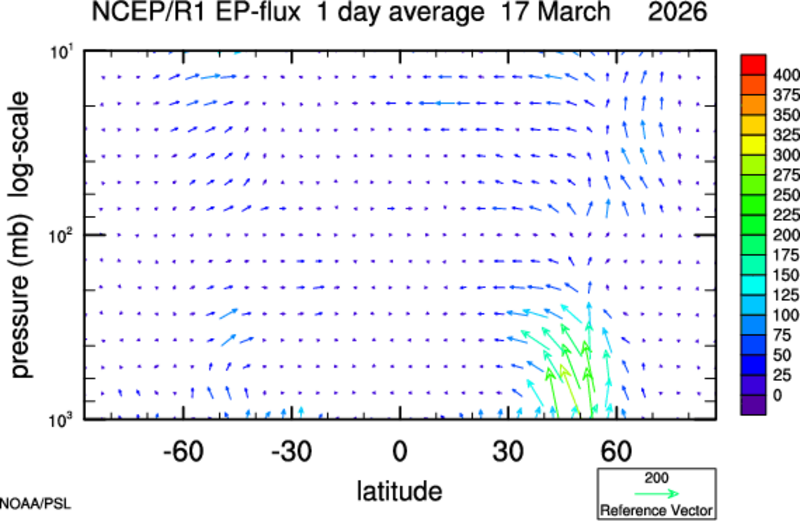# EP FluxesLast day available Nov 26, 2023
 Plot either the EP Flux averaged from daily data over N days until the date you select. Data is taken from the NCEP/NCAR Reanalysis which begins Jan 1 1948.
Animation | Pregenerated Plots for recent months

 Using Daily Data: Date: YYYY MM DD (latest date Nov 26, 2023 ) Averaging Period 1 day 7 days 10 days 14 days 30 days Stratospheric Scale Factor: 1 2 3 4 5 6 7 8 9 10 Calculate Convergence/Divergence* EP Flux Vectors Only Vectors and Divergence Vectors and Acceleration Scale by Square Root** On Off

Quasi-geostrophic Eliassen Palm flux on the sphere are computed from daily-averaged values of wind and temperature taken from the NCAR/NCEP Reanalysis hosted at ESRL/PSL. The EP-Flux vectors have been scaled for display purposes. Contours show the value of EP Flux divergence. Areas of EP-Flux convergence, and hence of westerly deceleration shown by solid lines. Note that vectors above 100mb will be multiplied by a scale factor which you can change.

*Calculate divergence

Setting this to "On" will calculate and plot contours of the EP-Flux divergence on the same plot as the EP Flux vectors. "Off" will only plot the EP Flux vectors. "Show Acceleration". Plots the acceleration of the zonal wind instead of the divergence of EP Flux. The difference between the two is a factor of a*cos(latitude).
**Scale by Square Root
Scales the EP Flux vectors for display by a factor proportional to the square-root of pressure. This is done in order to enhance visibility of vectors in the stratosphere.

This is a Research and Development Application